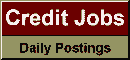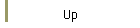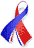DefaultRisk.com the web's biggest credit risk modeling resource.Export citation to:- HTML- Text (plain)- BibTeX- RIS- ReDIF

Tail Approximations for Portfolio Credit Risk

by Paul Glasserman of Columbia Business School

October 2004

Abstract: This paper develops approximations for the distribution of losses from default in a normal copula framework for credit risk. We put particular emphasis on approximating small probabilities of large losses, as these are the main requirement for calculation of value at risk and related risk measures. Our starting point is an approximation to the rate of decay of the tail of the loss distribution for multifactor, heterogeneous portfolios. We use this decay rate in three approximations: a homogeneous single-factor approximation, a saddlepoint heuristic, and a Laplace approximation. The first of these methods is the fastest, but the last two can be surprisingly accurate at very small loss probabilities. The accuracy of the methods depends primarily on the structure of the correlation matrix in the normal copula. Calculation of the decay rate requires solving an optimization problem in as many variables as there are factors driving the correlations, and this is the main computational step underlying these approximations. We also derive a two-moment approximation that fits a homogeneous portfolio by matching the mean and variance of the loss distribution. Whereas the complexity of the other approximations depends primarily on the number of factors, the complexity of the two-moment approximation depends primarily on the size of the portfolio.

Published in: Journal of Derivatives, Vol. 12, No. 2, (Winter 2004), pp. 24-42.

Books Referenced in this paper:  (what is this?)